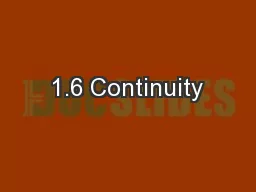# 1.6 ContinuityEmbed code:

## 1.6 Continuity

Download Presentation - The PPT/PDF document "1.6 Continuity" is the property of its rightful owner. Permission is granted to download and print the materials on this web site for personal, non-commercial use only, and to display it on your personal computer provided you do not modify the materials and that you retain all copyright notices contained in the materials. By downloading content from our website, you accept the terms of this agreement.

### Presentations text content in 1.6 Continuity

Slide1

1.6 Continuity

Assigned work: pg 51 #4adef, bcdf,7,8,10-13A continuous curve is a curve without breaks, holes or jumps. Usually if we talk about a curve being discontinuous it is at a specific point. These are discontinuous….    HOLE JUMP ASYMPTOTE

S. Evans

Slide2

1.6 Continuity

S. Evans

Conditions for Continuity:

must exist

must exist (i.e. LHL=RHL) (i.e. condition 1 = condition 2)Note: For all polynomials so all polynomials are continuous.

Slide3

1.6 Continuity

S. Evans

In the following examples state which condition fails:

cond

1 cond 2 cond 1 cond 3

Slide4

1.6 Continuity

When determining if a function is continuous you need to know where you should look for discontinuities…..For Rational functions: Look at when the denominator equals 0. If discontinuous, would fail condition 1 and 2If discontinuous, would be a hole or asymptote

S. Evans

Slide5

1.6 Continuity

For Piecewise Functions:Look at the extreme domain valuesIf discontinuous, could fail condition 1, 2, and/or 3.If discontinuous, would be a jump or hole.NOTE: We are fussy about format for continuity so show ALL conditions in your work when showing if function is continuous or not.

S. Evans

Slide6

1.6 Continuity

Rational Function Examples:Ex. 1:For what values of x is f(x) discontinuous. Show why, state which condition fails and what discontinuity you have.a)

S. Evans

Hole at x = -1

Asymptote at x = 2We need to show conditions where denominator is = 0 (see next slide)

Slide7

1.6 Continuity

S. Evans

So f(x) is discontinuous So f(x) is discontinuous

at x = -1 (hole at -1,-1/3) at x = 2 (vert. asymptote)

Slide8

1.6 Continuity

S. Evans

So f(x) is discontinuous at x = 5

(

vert. asymptote

)

b)

Asymptote at x = 5

Now show conditions

Slide9

1.6 Continuity

Piecewise Function Examples:Ex. 2:For what values of x is f(x) discontinuous. Show why, state which condition fails and what discontinuity you have.a)

S. Evans

Ask yourself where might f(x) be discontinuous? Look at domains – notice 1 & -1.

Slide10

1.6 Continuity

S. Evans

So f(x) is continuous So f(x) is discontinuous

at x = -1 at x = 1 (jump)

Slide11

1.6 Continuity

b)

S. Evans

Ask yourself where might f(x) be discontinuous? Look at

domains i.e. 0

So

f(x) is discontinuous at x= 0 (hole at 0,1)

Now Graph it